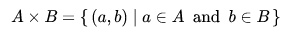﻿ Python: Cartesian product of two or more given lists using itertools - w3resource

# Python: Cartesian product of two or more given lists using itertools

## Python Itertools: Exercise-12 with Solution

Write a Python program to compute the Cartesian product of two or more given lists using itertools.

In mathematics, specifically set theory, the Cartesian product of two sets A and B, denoted A × B, is the set of all ordered pairs (a, b) where a is in A and b is in B. In terms of set-builder notation, that isA table can be created by taking the Cartesian product of a set of rows and a set of columns. If the Cartesian product rows × columns is taken, the cells of the table contain ordered pairs of the form (row value, column

Sample Solution:

Python Code:

``````import itertools
def cartesian_product(lists):
return list(itertools.product(*lists))

ls = [[1,2],[3,4]]
print("Original Lists:",ls)
print("Cartesian product of the said lists: ",cartesian_product(ls))
ls = [[1,2,3],[3,4,5]]
print("\nOriginal Lists:",ls)
print("Cartesian product of the said lists: ",cartesian_product(ls))
ls = [[],[1,2,3]]
print("\nOriginal Lists:",ls)
print("Cartesian product of the said lists: ",cartesian_product(ls))
ls = [[1,2],[]]
print("\nOriginal Lists:",ls)
print("Cartesian product of the said lists: ",cartesian_product(ls))
```
```

Sample Output:

```Original Lists: [[1, 2], [3, 4]]
Cartesian product of the said lists:  [(1, 3), (1, 4), (2, 3), (2, 4)]

Original Lists: [[1, 2, 3], [3, 4, 5]]
Cartesian product of the said lists:  [(1, 3), (1, 4), (1, 5), (2, 3), (2, 4), (2, 5), (3, 3), (3, 4), (3, 5)]

Original Lists: [[], [1, 2, 3]]
Cartesian product of the said lists:  []

Original Lists: [[1, 2], []]
Cartesian product of the said lists:  []
```

Python Code Editor:

Have another way to solve this solution? Contribute your code (and comments) through Disqus.

What is the difficulty level of this exercise?

Test your Programming skills with w3resource's quiz.

﻿